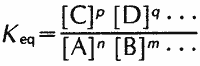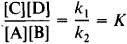chemical equilibrium

(redirected from Equilibrium reaction)
Also found in: Dictionary, Thesaurus, Medical.
Related to Equilibrium reaction: chemical equilibrium

equilibrium, chemical:

see chemical equilibriumchemical equilibrium,
state of balance in which two opposing reversible chemical reactions proceed at constant equal rates with no net change in the system. For example, when hydrogen gas, H2, and iodine gas, I2
.

chemical equilibrium,

state of balance in which two opposing reversible chemical reactionschemical reaction,
process by which one or more substances may be transformed into one or more new substances. Energy is released or is absorbed, but no loss in total molecular weight occurs.
proceed at constant equal rates with no net change in the system. For example, when hydrogen gas, H2, and iodine gas, I2, are mixed, and gaseous hydrogen iodide, HI, is formed according to the equation H2 + I2 → 2HI, no matter how long the reaction is allowed to proceed some quantity of hydrogen and iodine will remain unreacted. The reason reactants in a reversible reaction are never completely converted to product is that an opposing reaction is taking place simultaneously, i.e., some of the newly formed HI is being converted back into hydrogen and iodine. For any particular temperature, a point of equilibrium is reached at which the rates of the two opposing reactions are equal and there is no further change in the system. This equilibrium point is characterized by specific relative concentrations of reactants and products and will also be reached from the opposite direction, i.e., if one starts with hydrogen iodide and allows it to decompose into hydrogen and iodine. The equilibrium point can be described by the mass action expression, which defines the equilibrium constant, Keq, in terms of the ratio of the molar concentrationsconcentration,
in chemistry, measure of the relative proportions of two or more quantities in a mixture. The concentration of a solute is very important in studying chemical reactions because it determines how often molecules collide in solution and thus indirectly determines
of the products to those of the reactants. For the reversible reaction used as an example, the equilibrium constant is Keq=[HI]2/[H2][I2]; for the general reversible reaction nA + mB + · · · ⇋ pC + qD + · · · , the equilibrium constant is:where [A], [B], [C], [D], … are the molar concentrations of the substances and n, m, p, q, … are the coefficients of the balanced chemical equation. The larger the equilibrium constant for a given reaction, the more the reaction is favored, since a larger value of Keq means larger concentrations of the products relative to the reactants. The equilibrium constant is related to the change in the standard free energy, G°, of the system by the equation ΔG° = −RT. ln Keq, where R is a constant, T is the temperature in degrees Kelvin, and ln Keq is the natural logarithm of the equilibrium constant. Chemical equilibrium can be defined for many types of chemical processes, such as dissociationdissociation,
in chemistry, separation of a substance into atoms or ions. Thermal dissociation occurs at high temperatures. For example, hydrogen molecules (H2
of a weak acid in solution, solubility of slightly soluble salts, and oxidation-reduction reactions. In all of these cases, the equilibrium constant or its analogue is defined for certain conditions of temperature and other factors. If any of these factors change, the system will respond to establish a new equilibrium, in accordance with Le Châtelier's principleLe Châtelier's principle,
chemical principle that states that if a system in equilibrium is disturbed by changes in determining factors, such as temperature, pressure, and concentration of components, the system will tend to shift its equilibrium position so as to
.

Equilibrium, Chemical

the state of a system in which one or more reversible chemical reactions occur and in which the forward and reverse rates for each reaction are equal. The composition of the system therefore remains constant so long as the conditions of its existence are preserved. In the simplest case, given a homogeneous system in which a reversible chemical reaction

A + B ⇄ C + D

occurs, the rate of the forward reaction is proportional to the concentration of reactants:

v1 = k1[A][B]

The rate of the reverse reaction is proportional to the concentration of reaction products:

v2 = k2[C][D]

where k1 and k2 are the corresponding rate constants under the given conditions. At the initial moment, when [C] and [D] are equal to zero, v2 = 0 and v1 is determined by the initial concentrations of A and B. Rate vl decreases as A and B are consumed, while v2 increases as C and D are formed. Eventually, the rates become equal (v1 = v2); that is, chemical equilibrium is established. From the equality v1 = v2 it follows thatwhere [C], [D], [A], and [B] are the equilibrium concentrations of the substances and K is the equilibrium constant, whose value for each reversible reaction is dependent on external conditions. The relationship thus obtained expresses the law of mass action, which denotes the extent to which the original composition of a system can change through a spontaneous reaction under given conditions, that is, without any work being done on the system from without. Under conditions of chemical equilibrium, the concentrations (activity) of all the substances are interrelated; it is impossible to change one without changing all the others. The given expression for K holds for gaseous reactions at low pressures and for dilute solutions.

Thermodynamically, chemical equilibrium for both homogeneous and heterogeneous systems is defined as the most stable state under given conditions; that is, it is one in which, depending on the method of assigning external conditions, one or another thermodynamic function of the state reaches its minimum or maximum value. For isolated systems, those that exchange neither matter nor energy with the external medium, this function is called entropy. In the equilibrium state, the entropy is at its maximum. If heat exchange is possible with the surrounding medium but the temperature and pressure in the system are constant, then the isobaric-isothermal potential is at its minimum (see GIBBS FREE ENERGY). In the case of constant temperature and volume, the isochoric-isothermal potential will assume its minimum value (seeHELMHOLTZ FREE ENERGY).

The dependence of chemical equilibrium on external conditions is expressed qualitatively by the Le Châtelier-Braun principle and quantitatively by the corresponding thermodynamic equations. For example, the effect of temperature is expressed by the equations for the isobar or isochor of the reaction.

The study of chemical equilibrium has great theoretical and practical importance, expecially when applied to processes that occur in complex systems. The considerable difficulties encountered when experimental methods are used in the study of chemical reactions at elevated temperatures (high-temperature chemistry) have led to the development of calculations for equilibrium mixture compositions under given initial external conditions and given initial concentrations (or quantities) of components. In chemical technology, determining the position of chemical equilibrium at various pressures and temperatures and taking into account the reaction rates enable the selection of optimum process conditions, in particular, the conditions favoring the maximum yield of chemical products. The calculation of the initial composition of mixtures has become extremely important, as has that of the composition of quasi-equilibrium systems, in which one or more possible thermodynamic reactions practically do not occur or else proceed very slowly by virtue of their specific kinetic properties.

REFERENCES

Kurs fizicheskoi khimii, 2nd ed., vol. 1. Editor in chief, Ia. I. Gerasimov. Moscow, 1969.
Termodinamicheskie i teplofizicheskie svoistva produktov sgoraniia. Spravochnik, vol. 1. Moscow, 1971.

M. E. ERLYKINA

chemical equilibrium

[′kem·i·kəl ‚ē·kwə′lib·rē·əm]
(chemistry)
A condition in which a chemical reaction is occurring at equal rates in its forward and reverse directions, so that the concentrations of the reacting substances do not change with time. Also known as equilibrium.
References in periodicals archive ?
Remaining equations are combined to a single chemical equilibrium reaction shown in Eq.
With multiple niches, this equilibrium reaction norm may be far from the corresponding set of optimal values.
As the silanization is an equilibrium reaction, lower concentrations of ethanol in the compound were expected to increase the reaction rate.
A wet silica treatment process initially seemed very inefficient because we expected the excess water to keep this equilibrium reaction from working.
Both groups could have measurements taken of muscle activation to determine if righting reactions or equilibrium reactions are being utilized.
Vinal, A Study of Equilibrium Reactions in the Lechlanche Dry Cells, Trans.

Site: Follow: Share:
Open / Close